#### 期刊菜单

Study on the Evaluation Method of Division Factor of Grounding Engineering in Adverse Geological Environment
DOI: 10.12677/JEE.2021.91006, PDF, HTML, XML, 下载: 229  浏览: 579

Abstract: There is a problem of high grounding resistance in the grounding engineering under the harsh geological environment, which is easy to lead to high ground potential in the case of fault, endan-gering the safe operation of the system and personal safety. At present, the calculation of fault current distribution and shunt coefficient is usually limited to the simple case of single circuit overhead line or underground cable, and the coupling effect of non fault phase is not considered in most cases, and the influence of transformer is not considered in the model. To solve this problem, the theoretical model of short-circuit current to ground is deduced theoretically, and different situations are distinguished according to different fault locations. Secondly, based on EMTP electromagnetic transient program, a simplified calculation scheme of ground current is proposed, and the method is applied to a hydropower station with adverse geological environment. The results show that the grounding short-circuit current refers to the part of the short-circuit current which constitutes the grounding grid earth circuit and then causes the grounding grid ground potential to rise. The calculation needs to consider the maximum grounding short-circuit fault current that may occur in four cases, namely, the short-circuit inside the station, the short-circuit outside the station, the single-phase grounding short-circuit fault and the two-phase grounding short-circuit fault. This method is helpful to calculate the short-circuit current quickly and accurately, and provides an effective reference for accurate calculation of grounding parameters.

1. 引言

2. 入地短路电流计算方法

2.1. 分流系数的简易计算法

$\frac{1}{Z}=\frac{1}{{R}_{2}}+\frac{1}{{Z}_{1}+Z}$ (1)

$Z=-\frac{{a}_{\text{1}}+j{b}_{\text{1}}}{2}+\frac{1}{2}\sqrt{\left({a}_{1}^{2}-{b}_{1}^{2}+4{a}_{1}{R}_{1}\right)+j\left(2{a}_{1}{b}_{1}+4{b}_{1}{R}_{2}\right)}$ (2)

$Z=a+jb$ (3)

$\frac{1}{{Z}_{0}}=\frac{1}{{R}_{1}}+\frac{1}{{Z}_{1}+Z}$ (4)

${Z}_{\text{1}}={a}_{\text{1}}+j{b}_{\text{1}}$$Z=a+jb$ 带入(4)式，整理后有：

${Z}_{0}=\frac{{R}_{1}\left({a}_{1}+a\right)+j{R}_{1}\left({b}_{1}+b\right)}{\left({R}_{1}+{a}_{1}+a\right)+j\left({b}_{1}+b\right)}={a}_{0}+j{b}_{0}$ (5)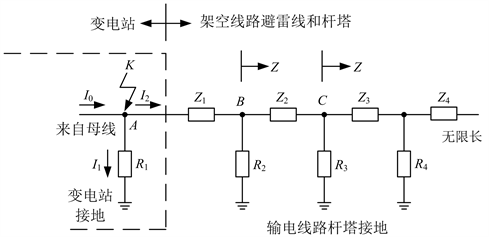Figure 1. The equivalent network diagram of the shunting of the “lightning wire-tower” grounding system

2.2. 分流系数的直接公式法

${I}_{g1}=\left({I}_{\mathrm{max}}-{I}_{n}\right){K}_{f1}$ (6)

${K}_{f1}=1-\left[\frac{{\text{e}}^{\beta s}-{\text{e}}^{-\beta s}}{{\text{e}}^{\beta \left(s+1\right)}-{\text{e}}^{-\beta \left(s+1\right)}}\left(1-\frac{{Z}_{m}}{{Z}_{s}}\right)+\frac{{Z}_{m}}{{Z}_{s}}\right]$ (7)

${\text{e}}^{\beta }=\frac{1-\sqrt{\frac{{Z}_{s}D}{12{R}_{st}+{Z}_{s}D}}}{1+\sqrt{\frac{{Z}_{s}D}{12{R}_{st}+{Z}_{s}D}}}$ (8)

${Z}_{s}=\frac{3{r}_{s}}{k}+0.15+j0.189\mathrm{ln}\frac{{D}_{g}}{\sqrt[k]{{\alpha }_{s}{D}_{s}^{k-1}}}$ (9)

${Z}_{m}=0.15+j0.189\mathrm{ln}\frac{{D}_{g}}{{D}_{m}}$ (10)

${D}_{m}=\sqrt{{D}_{1A}{D}_{1B}{D}_{1C}}$ (11)

${D}_{m}=\sqrt{{D}_{1A}{D}_{1B}{D}_{1C}{D}_{2A}{D}_{2B}{D}_{2C}}$ (12)

${D}_{g}=80\sqrt{\rho }$ (13)

${K}_{f1}=1-{\text{e}}^{-\beta }\left(1-\frac{{Z}_{m}}{{Z}_{s}}\right)-\frac{{Z}_{m}}{{Z}_{s}}$ (14)

2.3. 站内短路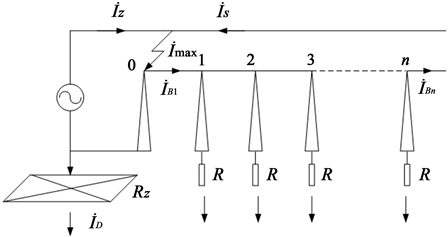Figure 2. The situation where a short circuit occurs in the grounding grid

${\stackrel{˙}{I}}_{B1}=\left({\stackrel{˙}{I}}_{\mathrm{max}}-{\stackrel{˙}{I}}_{Z}\right){K}_{f1}={\stackrel{˙}{I}}_{S}{K}_{f1}$ (15)

${\stackrel{˙}{I}}_{D}=\left({\stackrel{˙}{I}}_{\mathrm{max}}-{\stackrel{˙}{I}}_{Z}\right)\left(1-{K}_{f1}\right)$ (16)

${K}_{f1}=\frac{{\stackrel{˙}{I}}_{B1}}{{\stackrel{˙}{I}}_{S}}=\frac{{\stackrel{˙}{I}}_{B1}}{{\stackrel{˙}{I}}_{B1}+{\stackrel{˙}{I}}_{D}}=\frac{{\stackrel{˙}{I}}_{\mathrm{max}}-{\stackrel{˙}{I}}_{Z}-{\stackrel{˙}{I}}_{D}}{{\stackrel{˙}{I}}_{\mathrm{max}}-{\stackrel{˙}{I}}_{Z}}$ (17)

2.4. 站外短路

${\stackrel{˙}{I}}_{D}={\stackrel{˙}{I}}_{Z}\left(1-{K}_{f2}\right)$ (18)

${K}_{f2}=\frac{{\stackrel{˙}{I}}_{BS}}{{\stackrel{˙}{I}}_{Z}}=\frac{{\stackrel{˙}{I}}_{BS}}{{\stackrel{˙}{I}}_{BS}+{\stackrel{˙}{I}}_{D}}=\frac{{\stackrel{˙}{I}}_{Z}-{\stackrel{˙}{I}}_{D}}{{\stackrel{˙}{I}}_{Z}}$ (19)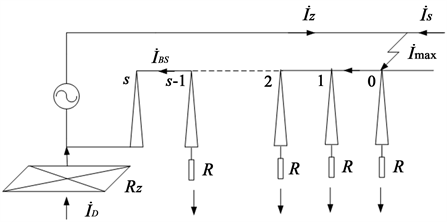Figure 3. The situation where a short circuit occurs outside the grounding grid

3. EMTP-ATP计算方法

EMTP电磁暂态程序具有计算暂态和稳态的功能，且计算速度快、精度高，利用EMTP程序可以避免过多的假设而造成的计算误差。根据零序等值网络和线路结构、参数，可利用EMTP程序分别对站内、站外发生单相接地短路时两种情况下的分流系数进行计算，研究短路电流和分流系数随变电站接地网接地电阻、杆塔接地电阻、避雷线型号、站外短路点位置等变化的规律，所以本文介绍采用ATP-EMTP对电站入地短路电流进行计算的方法。

3.1. EMTP-ATP基本算法

EMTP (Electromagnetic Transients Program)是道梅尔–白日朗于20世纪50年代末在美国BPA电力公司工作时编制的程序，ATP (Alternative Transient Program)是其Windows版本，是EMTP的继续和发展，它保存了EMTP的全部功能，还添加了一些新的功能，采用了与用户友好的人机界面，且ATP程序中的模型满足我们计算所需的各种电气设备的模型，并且很容易建立一些特殊的元件，计算精度也合适。其基本算法为：根据元件的不同特性，建立相应的代数方程，常微分方程和偏微分方程，利用梯形积分法将电感、电容、电源等集中参数元件化成电阻性网络，对于传输线等分布性参数利用其上的波过程的特征线方程，经过一定的转换，把分布参数的线段也等效为电阻性网络，则其相应的方程也变为代数方程，进一步形成节点导纳矩阵；然后采用优化结点编号技术和稀疏矩阵算法，以节点电压为未知量，利用矩阵三角分解求解，最后求得各支路的电流，电压和所有消耗的功率，能量。在稳态计算中应将非线性元件线性化，包括利用简单的迭代进行潮流计算。在暂态计算中非线性特性可以用分线段线性化来处理，也可进行迭代求解(即补偿法，就是将线性网络部分和非线性网络部分分开处理，使求解非线性电路的迭代计算限制在小部分网络中)。

ATPDraw目前支持大约70个标准组件和28个TACS对象。ATPDraw程序有标准的视窗平台，用户可以任意选择已经定义好的组件的模型(图形)来构造需要的电路，也可以自己定义不同的组件。然后由处理器自动生成相应的正确的ATP输入文件。支路的节点名可以由程序给定，也可以自定义。因此，基于图形化界面的ATPDRAW在使用中是极其方便和准确的。

ATP 程序和ATPDraw程序的综合运用，使得计算电磁暂态现象方面的问题更加方便、准确。其权威性和通用性强，求解速度快；精度能满足工程计算的要求；Windows人机对话界面，计算模型图形化输入，操作方便，计算结果得到业界普遍认可。

3.2. EMTP-ATP计算模型建立

1) 电压源模型

2) 变压器模型

ATP中变压器模型有单相或三相理想变压器模型、单相或三相饱和变压器模型、三相铁心式高对称阻抗饱和变压器模型和BCTRAN支持子程序。根据实际给出的变压器铭牌参数情况，计算中采用BCTRAN支持子程序，可以模拟各种绕组连接方式的变压器。

BCTRAN支持子程序可根据变压器在额定频率下空载和短路试验的数据，得到单相或三相，双绕组、三绕组或多绕组变压器的线性L−1、R或R、ωL矩阵表达式，表示变压器一次、二次各相之间的耦合。短路电阻矩阵为对角矩阵，对角元素分别和各绕组的电阻对应，铁耗等效电阻和各线圈并联。该模型需要空载励磁和短路试验数据作为输入参数。

3) 输电线路模型

4) 短路模型

${R}_{h}=1050\left({l}_{h}/{I}_{h}\right)\left(\Omega \right)$ (20)

4. 计算案例

4.1. 计算条件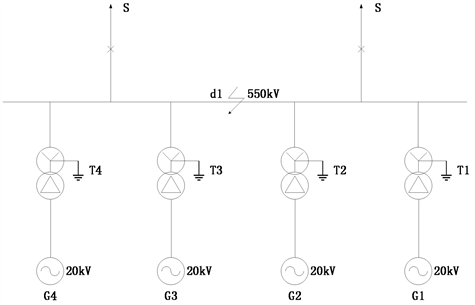Figure 4. Schematic diagram of the location of voltage and current lines

4.2. 参数选择

1) 等值电压源

${X}_{s1}=\frac{{S}_{n}}{\sqrt{3}{U}_{n}{I}_{k}}=\frac{100}{\sqrt{3}×525×21.4}=0.0051$

${X}_{s0}=\frac{{S}_{n}}{\sqrt{3}{U}_{n}{I}_{k}}×3-2×{X}_{s1}=\frac{100}{\sqrt{3}×525×21.7}×3-2×0.0051=0.0049$

2) 发电机

3) 变压器

4) 出线段线路

4.3. 输入参数

1) 基准电压

2) 等值电压源模型

${X}_{S1}=0.0051×\frac{{525}^{2}}{100}=14.0569\left(\Omega \right)$ (正序)

${X}_{S0}=0.0049×\frac{{525}^{2}}{100}=13.5056\left(\Omega \right)$ (零序)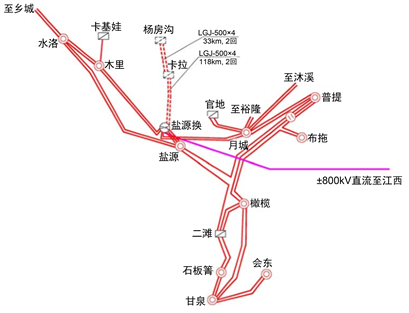Figure 5. Schematic diagram of hydropower station system wiring

${X}_{L1}=1.647\left(\Omega \right)$ (正序)

${X}_{L0}=7.945\left(\Omega \right)$ (零序)

${X}_{s1}=14.0569-1.647=12.4099\left(\Omega \right)$ (正序)

${X}_{s0}=13.5056-7.945=5.5606\left(\Omega \right)$ (零序)

3) 发电机模型

${X}_{G}={{X}^{″}}_{d}×\frac{{U}_{b}^{2}}{{S}_{G}}=0.214×\frac{{22.6}^{2}}{375}×0.9=0.26233\left( \Omega \right)$

4) 变压器模型

5) 高压并联电抗器

${X}_{L}=\frac{{U}_{n}^{2}}{{Q}_{L}}=\frac{{525}^{2}}{180}=1531\left( \Omega \right)$

6) 其它模型

4.4. 计算结果

1) 站内短路Table 1. Calculation results of short-circuit current when short-circuit in the station (kA) (power station grounding impedance 0.548 Ω)

2) 站外短路

IS为系统提供的短路电流，IBS为线路–杆塔接地系统分走的电流，ID为入地短路电流， ${K}_{f2}=\frac{{I}_{BS}}{{I}_{z}}=\frac{{I}_{BS}}{{I}_{BS}\text{+}{I}_{D}}$ 为分流系数。Table 2. Calculation results of short-circuit current when short-circuit outside the station (kA) (station grounding impedance 0.548 Ω)Table 3. The relationship between the ground short-circuit current and the position of the short-circuit point (two-phase short-circuit)

3) 最大入地电流Table 4. Calculation results of short-circuit current when two-phase short-circuit in the station (kA) (power station grounding impedance 1.849 Ω)

5. 结论

1) 恶劣地质环境下电站的接地分流系数评估需要考虑站内故障和站外故障两种情况。无论是何种形式的故障均要考虑非故障相的耦合作用。由于不能考虑电站接地电阻的变化以及多条线路之间故障相与非故障相之间的耦合，传统的简化公式法不适用于恶劣地质环境下电站的接地分流系数评估。而使用电磁暂态仿真程序建立相应的分流系数评估模型可以弥补简化公式法的缺陷。

2) 运用某恶劣地质环境地区电站的案例验证了方法的有效性，并分析了不同情况下的短路电流分布。结果表明入地短路电流的计算需要考虑站内短路和站外短路，以及单相接地短路故障和两相接地短路故障共四种情况下可能出现的最大入地短路故障电流。针对站外故障的情况，随着短路点离电站距离的增大，站内提供的短路电流逐渐减小，但系统侧提供的短路电流不断增大，入地短路电流呈现先增大后减小的趋势。所以在在外故障分析的过程当中要选取多个故障点的位置以确认电站入地电流的最大值。

  Substations Committee of the IEEE Power Engineering Society (2000) IEEE Guide for Safety of AC Substation Groundings. IEEE, New York.  中国电力企业联合会. GB 50065-2011交流电气装置的接地设计规范[S]. 北京: 中国计划出版社, 2011.  何金良, 曾嵘. 电力系统接地技术[M]. 北京: 科学出版社, 2007.  Deri, A., Tevan, G., Semlyen, A. and Castanheira, A. (1981) The Complex Ground Return Plane a Simplified Model for Homogeneous and Multi-Layer Earth Return. IEEE Transactions on Power Apparatus and Systems, PAS-100, 3686-3693. https://doi.org/10.1109/TPAS.1981.317011  多梅尔, 著. 电力系统电磁暂态计算理论[M]. 李永庄, 等, 译. 北京: 水利电力出版社, 1991.  Sunde, E.D. (1968) Earth Conduction Effects in Transmission Systems. Dover Publications, Inc., Mineola.  李谦编著. 电力系统接地网特性参数测量与应用[M]. 北京: 中国电力出版社, 2013.  国家能源局. DL∕T 475-2017 接地装置特性参数测量导则[S]. 北京: 中国计划出版社, 2019.  舒廉甫. 三峡左岸电站发电前枢纽接地装置接地电阻测量[C]//中国电机工程学会. 中国电机工程学会高电压专业委员会学术会议: 第一卷, 2004: 465-469.  方瑜. 四极法测量地网接地电阻的分析[J]. 高电压技术, 1989, 11(4): 41-44.  解广润. 电力系统接地技术[M]. 北京: 中国电力出版社, 1991.  李汝彪, 邱毓昌. 用附加串联电阻法消除接地电阻测量中的互感影响[J]. 中国电力, 1994(4): 64-66.  端木林楠, 赵习静, 蔡巍, 等. 变电站地网接地阻抗测量中互感消除方法研究[J]. 高压电器, 2014, 51(11): 140-145.  黄新波, 姬林垚, 朱永灿, 等. 电流极与电压极的引线夹角对接地电阻测量精度的影响研究[J]. 高压电器, 2017, 53(5): 14-21.  马御棠, 张博成, 周仿荣, 等. 消除引线互感影响的地网接地阻抗测量方法[J]. 电瓷避雷器, 2017(4): 73-77.  袁培, 何智强, 胡晓晖, 等. 考虑大地影响的直线法测量大型地网的接地阻抗[J]. 中国电力, 2018, 51(7): 36-42.  侯苏洋, 郝婷婷, 于洋, 等. 引线间互感对接地阻抗测量结果的影响分析[J]. 电瓷避雷器, 2019, 289(3): 52-56, 62.  Dawalibi, F. and Niles, G.B. (1984) Measurements and Computations of Fault Current Distribution on Overhead Transmission Lines. IEEE Transactions on Power Apparatus and Systems, PAS-103, 553-560. https://doi.org/10.1109/TPAS.1984.318744  Dawalibi, F. (1980) Ground Fault Current Distribution between Soil and Neutral Conductors. IEEE Transactions on Power Apparatus and Systems, PAS-99, 452-461. https://doi.org/10.1109/TPAS.1980.319679  Dawalibi, F., Bensted, D. and Mukhedkar, D. (1981) Soil Effects on Ground Fault Currents. IEEE Transactions on Power Apparatus and Systems, PAS-100, 3442-3450. https://doi.org/10.1109/TPAS.1981.316687  Gooi, H.B. and Sebo, S.A. (1985) Distribution of Ground Fault Currents along Transmission Lines—An Improved Algorithm. IEEE Transactions on Power Apparatus and Systems, 104, 663-670. https://doi.org/10.1109/TPAS.1985.319002  Garrent, D.L., Myers, J.G. and Patel, S.G. (1987) Determination of Maximum Substation Grounding System Fault Current Using Graphical Analysis. IEEE Transactions on Power Delivery, 2, 725-732. https://doi.org/10.1109/TPWRD.1987.4308170  IEEE Std.80-2000 (2000) Guide for Safety in AC Substation Grounding. Substations Committee of the IEEE Power Engineering Society, New York.  Sarkar, T.K. and Pereira, O. (1995) Using the Matrix Pencil Method to Estimate the Parameters of a Sum of Complex Exponentials. IEEE Antennas and Propagation Magazine, 37, 48-55. https://doi.org/10.1109/74.370583  Sheshyekani, K., Karami, H.R., Dehkhoda, P., Paolone, M. and Rachidi, F. (2012) Application of the Matrix Pencil Method to Rational Fitting of Frequency-Domain Responses. IEEE Transactions on Power Delivery, 27, 2399-2408. https://doi.org/10.1109/TPWRD.2012.2208986  Heppe, A.J. (1979) Computation of Potential at Surface above an Energized Grid or Other Electrode, Allowing for Nonuniform Current Distribution. IEEE Transactions on Power Apparatus and System, PAS-98, 665-670. https://doi.org/10.1109/TPAS.1979.319377